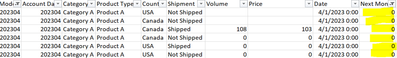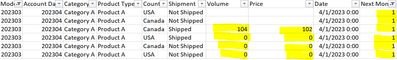cancel
Showing results for
Did you mean:Frequent Visitor

## Help with replacing Value on one Column based on Historical Values and another column

Hello,

I have a data where we are tracking figures for different models on monthly basis. These models are based on the month name. In my sample data here, Model column represents that and 202304 is the latest model and 202303 is the previous month's model and so on.

We are tracking forecasts on this table for next 24 months ahead. In this example, I am showing for next 3 months represented by column "Next Month" as 1, 2, and 3. 0 represents current month (202304) and -1, -2, -3, and so on represents previous months (limiting only to last 3 months for this example, however, it goes back to all historical values)

At the moment we are interested in replacing the current month's value ie where Next Month is 0 to the value of previous Model's next month forecast. So, for Model 202304 and for its Next Month Column at 0 and Account Date at 202304 should have values from previous model 202303 from its next month's forecast at 1 and Account Date at 202304. Ideally we would like to replace all the historical values where Next Month is 0, -1, -2, etc to its Previous Models of 202303, 202302, 202301 and so on. But for now, even if we can just replace current month's value at 0 to previous model's value, that would work for the time being.For instance, Above to be changed as follows:In Power BI, 202304 value in Matrix should 104 instead of 108Here the sums are coming right as well, but I having difficulty in getting right totals in my real data.

Regards,

KS

1 ACCEPTED SOLUTIONFrequent Visitor

Okay, I was able to solve this. Thanks!

2 REPLIES 2Frequent Visitor

Okay, I was able to get two measures with correct values.

FC just like I showed earlierAnd also AC with value 104 in 202304 and remaining empty cells in other columns

But in this table I can see one row for FC and another row for AC.

How can I combine the two measures or overlap the two measures that AC of 104 on 202304 column sits on FC of 202304? In other words, I would like to see just one row having FC Values in all columns except for 202304 and AC Value in that place within same row.

Any help to merge the two measures would be super helpful.

Thanks!Frequent Visitor

Okay, I was able to solve this. Thanks!